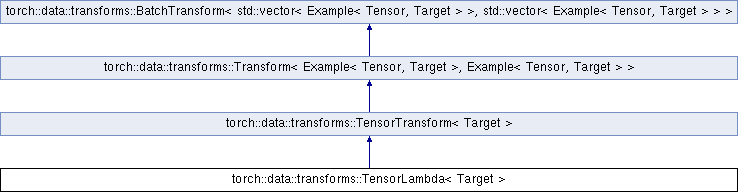Caffe2 - C++ API A deep learning, cross platform ML framework
torch::data::transforms::TensorLambda< Target > Class Template Reference

A `Lambda` specialized for the typical `Example<Tensor, Tensor>` input type. More...

`#include <tensor.h>`

Inheritance diagram for torch::data::transforms::TensorLambda< Target >:## Public Types

using FunctionType = std::function< Tensor(Tensor)>Public Types inherited from torch::data::transforms::TensorTransform< Target >
using E = Example< Tensor, Target >Public Types inherited from torch::data::transforms::Transform< Example< Tensor, Target >, Example< Tensor, Target > >
using InputType = Example< Tensor, Target >

using OutputType = Example< Tensor, Target >Public Types inherited from torch::data::transforms::BatchTransform< std::vector< Example< Tensor, Target > >, std::vector< Example< Tensor, Target > > >
using InputBatchType = std::vector< Example< Tensor, Target > >

using OutputBatchType = std::vector< Example< Tensor, Target > >

## Public Member Functions

TensorLambda (FunctionType function)
Creates a `TensorLambda` from the given `function`.

Tensor operator() (Tensor input) override
Applies the user-provided functor to the input tensor.Public Member Functions inherited from torch::data::transforms::TensorTransform< Target >
OutputType apply (InputType input) override
Implementation of `Transform::apply` that calls `operator()`.Public Member Functions inherited from torch::data::transforms::Transform< Example< Tensor, Target >, Example< Tensor, Target > >
std::vector< Example< Tensor, Target > > apply_batch (std::vector< Example< Tensor, Target > > input_batch) override
Applies the `transformation` over the entire `input_batch`.

## Detailed Description

### template<typename Target = Tensor> class torch::data::transforms::TensorLambda< Target >

A `Lambda` specialized for the typical `Example<Tensor, Tensor>` input type.

Definition at line 37 of file tensor.h.

The documentation for this class was generated from the following file:
• torch/csrc/api/include/torch/data/transforms/tensor.h